## Website-Einstellungen

##### Währungseinstellungen
Produkte
HF
EMI
Automobiltechnik
Informationsmaterial
Produktdokumentation
Handhabung und Verarbeitung
Modelle und Layout-Tools
Kurse/Schulung
Allgemein
Video-Bibliothek
Cx Family Common Mode Chokes
0402CT Low Profile Chip Inductors
XAL7050 High-inductance Shielded Power Inductors
XGL4020 Ultra-low DCR Power Inductors
Quality
Qualitätszertifizierung
Materialzertifizierung
Sicherheitszertifizierung
Zuverlässigkeit
Handhabung / Verarbeitung
Über
Über
Jobs
Standorte
Informationsmaterial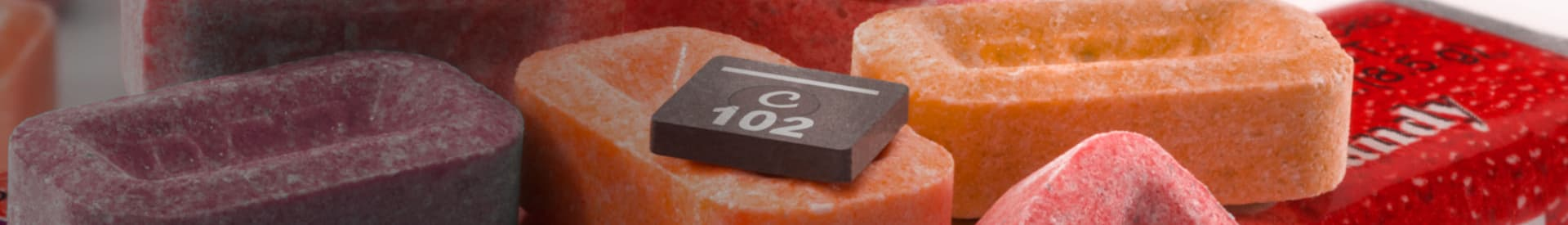# Using Coilcraft’s models in LTspice®

LTspice is a high performance SPICE simulator that simplifies the design of switching regulators. The software is provided free by Analog Devices. LTspice includes a library of basic models for a limited number of Coilcraft inductor models. These models are included in the standard inductor library and are updated periodically.

Coilcraft also offers an easy to install and use advanced model library that can be downloaded from the LTspice library page linked to the right. Installation and usage instructions for these libraries can be found on the same page.

### Model construction

The schematic below is the model structure of the Coilcraft advanced power inductor model. This may differ slightly between series and is different from the RF or Transmission line models.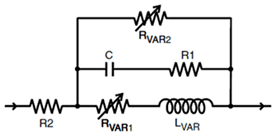Depending on the inductor series, the advanced SPICE models may include a variable inductor element, variable series resistance (Rvar1) to model skin effect, a resistor element in series with the parallel capacitance (R1) to limit impedance at resonance, and a variable parallel resistance (Rvar2) to model frequency-dependent low power core losses.

The schematic, element values, and coefficients for variable elements are provided in the PSPICE model document for each inductor series.

The GLaplace component is used in SPICE to describe a frequency-dependent impedance or resistance. Each frequency-dependent element (Rvar1, Rvar2, Lvar) is modeled in LTspice using the GLaplace G2 voltage dependent current source.

### Creating the Model in LTspice

• Place components for all fixed resistors and the capacitor.
• Change the values to those shown on the Coilcraft SPICE model document for the inductor being modeled.
• Place a Glaplace component for each variable resistor and inductor element (typically Rvar1, Rvar2, and Lvar).
• For each Gaplace component, copy and paste the corresponding Laplace statements below into the value field of the Glaplace element. (Be sure to rename L1 if multiple inductors are used).
```For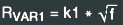use LAPLACE=+1/{L1_K1}/(-S*S/4/PI^2)^0.25
For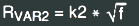use LAPLACE=+1/{L1_K2}/(-S*S/4/PI^2)^0.25
For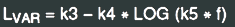use LAPLACE=+1/(S*1e-6*({L1_K3} - ({L1_K4}*LOG({L1_K5}*(abs(S)/(2*pi))))))```
• Next, use .param statement to define the K1 through K5 coefficients from the Coilcraft SPICE model document.
• Connect the components per the SPICE document schematic.

### Example Schematic and Netlist

The schematic below uses the Coilcraft XGL4020-102 as an example: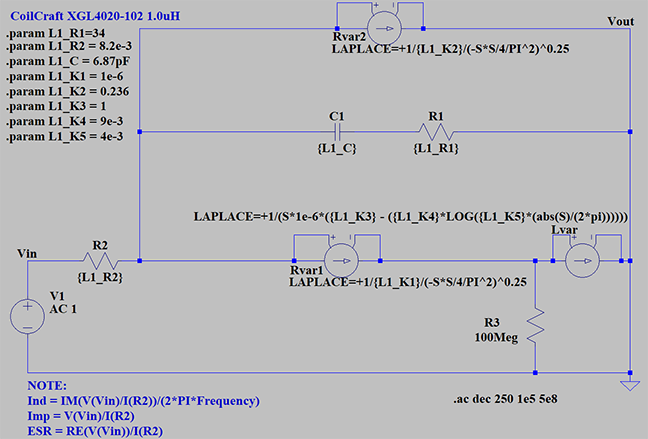This is the corresponding netlist for the example schematic:
```* Coilcraft XGL4020-102 1.0uH
V1 Vin 0 AC 1
R2 N001 Vin {L1_R2}
R1 0 N002 {L1_R1}
C1 N002 N001 {L1_C}
G§Rvar1 N001 0 N001 0 LAPLACE=+1/{L1_K2}/(-S*S/4/PI^2)^0.25
G§Rvar2 N001 N003 N001 N003 LAPLACE=+1/{L1_K1}/(-S*S/4/PI^2)^0.25
G§Lvar1 N003 0 N003 0 LAPLACE=+1/(S*1e-6*({L1_K3}-({L1_K4}*LOG({L1_K5}*(abs(S)/(2*pi))))))
R3 N003 0 100Meg
.param L1_R1 = 34
.param L1_R2 = 8.2e-3
.param L1_C= 6.87pF
.param L1_K1 = 1e-6
.param L1_K2 = 0.236
.param L1_K3=1
.param L1_K4 = 9e-3
.param L1_K5 = 4e-3
.ac dec 250 1e5 5e8
* NOTE: \nInd   = IM(V(Vin)/I(R2))/(2*PI*Frequency)\nImp  = V(Vin)/I(R2)\nESR= RE(V(Vin)/I(R2))
.backanno
.end
```

When running simulations, note the upper and lower frequency limits for which the model is valid. This information is shown on the Coilcraft SPICE model document for each inductor series.

### Plotting Waveforms

To plot Inductance vs frequency (best viewed in Cartesian form):
`Im(V(Vin)/I(R2))/(2*pi*frequency)`
To plot Impedance vs frequency (best viewed in Bode Logarithmic form):
`V(Vin)/I(R2)`
To plot ESR vs frequency (best viewed Bode Logarithmic form):
`Re(V(Vin)/I(R2))`
To plot Q factor vs frequency (best viewed in Cartesian form):
`ABS(Im(V(n001)/I(R2))/Re(V(n001)/I(R2)))`
*note voltage node names may be different it is up to user to ensure voltage is across inductor